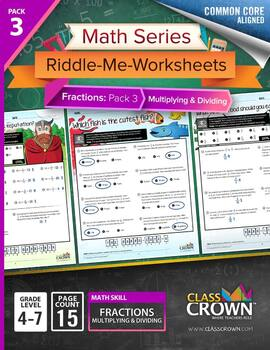Fractions Worksheets - Math Riddles - Pack 3 Multiply & Divide - 4th–7th Grade4th - 7th, Homeschool
Subjects
Resource Type
Formats Included
• PDF
Pages
26 pages

\$17.99
\$23.96
Save \$5.97

Description

Math Series: Riddle-Me-Worksheets

Fractions Pack 3 – Multiplying and Dividing

Do your students ever complete an entire worksheet, only to find that they have done most of the problems incorrectly and clearly have a misconception?

ClassCrown’s Riddle-Me-Worksheets help to prevent this problem by introducing a puzzle aspect to math, giving students immediate feedback as to whether or not they are solving problems correctly. If the answer to the riddle isn't spelled correctly, the student knows which problems he's made an error on.

This fractions pack contains skills covered in grades 4-7. While some of the included skills may be advanced for a younger student, the more difficult pages can be used as enrichment for advanced students. Each page has been designed in full, vibrant color for maximum quality but has been engineered to look great when printed in black and white.

Page Count: 15

Math Skill: Multiplying and Dividing Fractions

Skills Included:

• Multiplying whole numbers by fractions

• Multiplying whole number by fractions, word problems

• Representing multiplication of fractions visually

• Multiplying two fractions

• Multiplying two fractions, word problems

• Multiplying three fractions

• Multiplying a mixed number by a whole number

• Multiplying mixed numbers and fractions

• Multiplying two mixed numbers

• Dividing whole numbers by fractions

• Dividing fractions by whole numbers

• Dividing two fractions

• Dividing fractions and mixed numbers

• Dividing two mixed numbers

• Multiplying and dividing fractions and mixed numbers, word problems

Features:

• High Quality PDF format

• Full vibrant color pages that print great in black and white

• Common Core aligned

Feel free to let us know how we're doing in the Ratings & Comments section. Also, check us out on the web at www.classcrown.com and don't forget to follow us on twitter for updates and teacher information.

---------------------------------------------------------------------------------------

The content of this document is copyrighted by ClassCrown™.

The redistribution, resale, modification, or republication of any part of this document and its attachments is strictly prohibited. See terms of use below.

Personal Use: With your purchase, you are being granted permission to copy and print the digital work. All copyrights remain with © 2013 ClassCrown.

---------------------------------------------------------------------------------------

Total Pages
26 pages
Included
Teaching Duration
N/A
Report this Resource to TpT
Reported resources will be reviewed by our team. Report this resource to let us know if this resource violates TpT’s content guidelines.